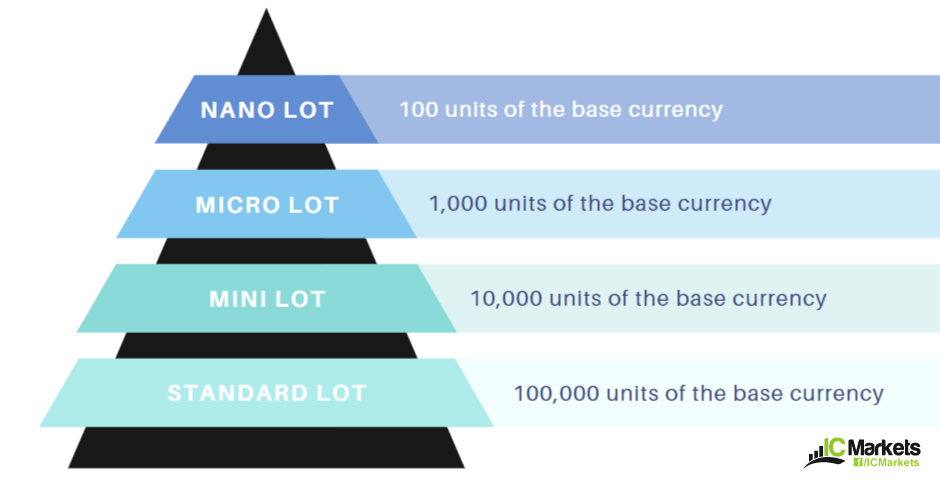# What Are Lots in the Forex Market?To trade the forex market successfully, understanding the dynamics behind how trades are measured is a necessity.

## Currency Pairs

A base currency is the primary currency in a currency pair quotation – the euro in EUR/USD, for example. The quote currency, or counter currency, is the US dollar, the second currency in the quotation.

Should you enter long (buy) EUR/USD, you buy the base currency and sell the counter currency; it’s always the base currency of the two currencies that is bought or sold. The quote currency is in place to determine the value of the base currency.

Some forex brokers display quantity in lots; others express size in currency units.

Trading lots vary between four key units.A standard lot is the equivalent to 100,000 units of the base currency.

A mini lot, 10% of a standard lot, is the equivalent to 10,000 units of the base currency.

A micro lot is the equivalent to 1,000 units of the base currency – popular with newer retail traders.

A nano lot is the equivalent to 100 units of the base currency, also common among newer traders with smaller account sizes.

The question then arises, is it necessary to have \$10,000 in the account to trade 1 mini lot? No. This is where leverage comes in.

## Leverage

Leverage is defined as having the ability to control larger sums of capital using little of your own funds. Contrary to popular belief, leverage in spot foreign exchange does not involve borrowing any money from the broker.

For every \$1 in your account you can control \$X amount where X is greater than 1. 100:1 leverage, for example, means you can control \$100 for each \$1 in your account. If you have \$1,000 in your account, you have the ability to control \$100,000 in positions.

You must also recognise what margin is. Margin is the amount of money a broker requires you to commit to cover potential future losses on a trade, before a position can be opened.

This article explores leverage in depth and highlights why leverage is not a loan in FX.

## Position Sizing

Position sizing is an important aspect, and can make or break a trader if not understood. Traders are risk managers, first and foremost.

Depending on the currency pair traded and account denomination, additional steps may be required.

Account denomination set the same as the counter currency:

Account currency: \$10,000

Currency pair: EUR/USD

Stop distance: 50 pips

Calculate risk to the account, which in this case is 2% (10,000 * 0.02 = \$200).

Divide the amount risked (\$200) by the stop-loss order distance to find the value per pip (\$200 / 50 = 4 [a value of 4\$ each pip]).

The final stage involves multiplying the pip value by the fixed unit value for EUR/USD.

If your trading account currency is in USD and the USD is listed as the second in a pair, the unit value of lots remain unchanged: \$0.10 per pip for a micro lot (1,000 units), \$1 per pip for a mini lot (10,000 units) and \$10 per pip for a standard lot (100,000 units).

Therefore, you can use the following calculation:

\$4 each pip * 10,000 units (mini lot) = 40,000 units of EUR/USD – 4 mini lots using a 50-pip stop distance.

Using a micro lot in the calculation, the position size is less, 4,000 units, or \$0.40 per pip, equating to \$20 risk over 50 pips.

Using a standard lot, trade risk is too high at 400k units, or \$40 per pip – a risk of \$2,000.

Account denomination set the same as the base currency:

Account currency: EUR 10,000

Currency pair: EUR/USD

Stop distance: 50 pips

Calculate risk to the account, which in this case is 2% (10,000 * 0.02 = EUR 200).

Convert the EUR value to USD as the value of a currency pair is calculated by the counter currency. The EUR/USD exchange rate, in this example, is \$1,1280.

Multiplying \$1.1280 by EUR 200, the trade risk, gives approximately \$225.60. This is the USD risk equivalent on the trade, as of the current exchange rate.

Next, divide the USD risk by the stop-loss distance, 50 pips: (USD 225.60) / (50 pips) = \$4.51 per pip.

Finally, multiply the value per pip by the lot value.

\$4.51 * (10,000/1) = approximately 45,000 units – 4 or 5 mini lots, depending on risk appetite. Or 4 mini lots and 5 micro lots. This is based on a 50-pip stop distance.

Account denomination differing from both the base and quote currency:

Account currency: USD 10,000

Currency pair: EUR/GBP

Stop distance: 50 pips

Calculate risk to the account, which in this case is 2% (10,000 * 0.02 = USD 200).

To find the correct position size, you need to find the value of risk in GBP. Remember, the value of a currency pair is in the counter currency. To convert the risk amount from USD to GBP, you need the GBP/USD exchange rate.

In this example, GBP/USD trades at 1.29011. You then multiply the trade risk \$200 by the inverted exchange rate (1 GBP / 1.29011 USD) = 154.00 risk in GBP.

Convert GBP risk to pip value by dividing by the stop-loss distance in pips: (GBP 154.00) / (50 pips) = GBP 3.08 per pip.

Finally, multiply the value per pip by the lot value:

3.08 * 10,000 = 30,800 units – approximately 3 mini lots.

Knowing the above, the trader can sell no more than 31,000 units of EUR/GBP, with a 50-pip stop distance, to stay within the pre-determined risk parameters set in USD.

## Forex Calculator

Make life easier.

While knowing the dynamics behind how trades are calculated is important, consider using our pip value calculator. This takes most of the leg work out of the calculation, giving you the freedom to focus on trading.